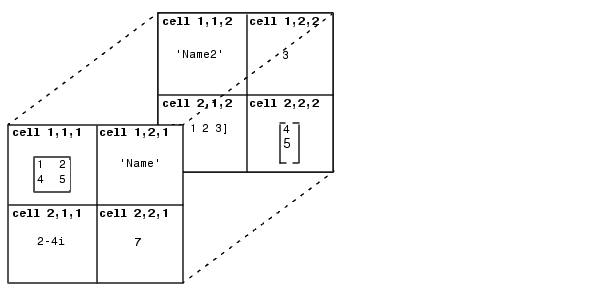ProgrammingMultidimensional Cell Arrays

Like numeric arrays, the framework for multidimensional cell arrays in MATLAB is an extension of the two-dimensional cell array model. You can use the `cat` function to build multidimensional cell arrays, just as you use it for numeric arrays.

For example, create a simple three-dimensional cell array `C`:

• ```A{1,1} = [1 2;4 5];
A{1,2} = 'Name';
A{2,1} = 2-4i;
A{2,2} = 7;
B{1,1} = 'Name2';
B{1,2} = 3;
B{2,1} = 0:1:3;
B{2,2} = [4 5]';
C = cat(3, A, B);
```

The subscripts for the cells of `C` look likeOrganizing Data in Multidimensional Arrays Multidimensional Structure Arrays© 1994-2005 The MathWorks, Inc.